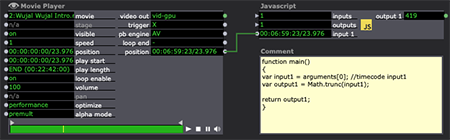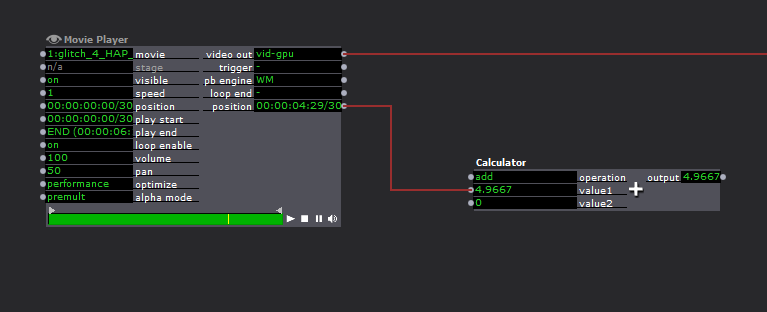# converting timecode to seconds

• Hi,

I am curious to know if the patch represented in this screengrab is the best approach for converting timecode to seconds?best wishes

Russell

• I found this javascript somewhere on the internetz and it can be modified to work with isdaora

```// set the frame rate or Frame Rat as we like to call him
var frameRat = 30 // fps
var numOfFrames = 60 // animation frame count
var animTime = "00:01:00:00" // time code
var a = convertTimeToFrames(animTime, frameRat);
var result = animTime + " at " + frameRat + " fps\n = " + a + " frames.";
alert(result);
function convertTimeCodeToSeconds(timeString, framerate)
{
var timeArray = timeString.split(":");  var hours   = parseInt(timeArray) * 60 * 60;  var minutes = parseInt(timeArray) * 60;  var seconds = parseInt(timeArray);  var frames  = parseInt(timeArray)*(1/framerate);  var str = "h:" + hours + "\nm:" + minutes + "\ns:" + seconds + "\nf:" + frames;  alert(str)  var totalTime = hours + minutes + seconds + frames;
//alert(timeString + " = " + totalTime)  return totalTime;
}
function convertTimeToFrames(timeString, framerate)
{  var secs = convertTimeCodeToSeconds(timeString, framerate);  return secs * framerate;
}```

• @fubbi said:

found this

👍 Thank you. The solution looks like it was much simpler than that. I assume there are built in converters at work here?```function main()
{
var input1 = arguments; //timecode input1
var output1 = Math.trunc(input1);
return output1;
}```

• To make things even easier, it should be possible to use Isadoras internal conversion for this.
Since isadora tracks timecode internally in milliseconds, you can treat timecode as an interger (seconds).
So this should provide the same conversion :)• @dusx

Thank' s a lot.

It is relative rare to find solutions here,

which are able to do without beeing a programmer.

R.h.

• @dusx said:

even easier

That couldn’t be easier. So timecode can be patched to both text and numeric inputs? Our job Is done here.